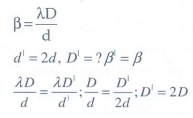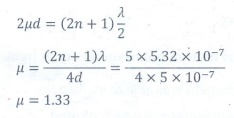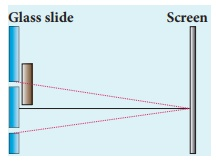# UNIT VII: Wave Optics - Online Test

Q1. A plane glass is placed over a various coloured letters (violet, green, yellow, red) The letter which appears to be raised more is,
Explaination / Solution:

The refractive index (n) of the glass is different for different colours. For violet n is high. ∴ Violet travel with low speed, so deviation is high.

Q2. Two point white dots are 1 mm apart on a black paper. They are viewed by eye of pupil diameter 3 mm approximately. The maximum distance at which these dots can be resolved by the eye is, [take wavelength of light, λ = 500 nm]
Explaination / Solution:

Resolution limit = 1.22 × λ / D

D - diameter of pupil = 3 mm

λ - wavelength = 500 nm

Resolution limit = distance between the dots / resolving diameter

= 1mm/d

[ 1.22 × λ ] / D =  1mm / d

D = [1mm × D ] / [ 1.22 × λ ] = [ 1 x 10-3 × 3 x 10-3 ] / [ 1.22 × 500 x10-9 ]

d = 4.918 ≈ 5m

Q3. In a Young’s double-slit experiment, the slit separation is doubled. To maintain the same fringe spacing on the screen, the screen-to-slit distance D must be changed to,
Explaination / Solution:Q4. Two coherent monochromatic light beams of intensities I and 4I are superposed. The maximum and minimum possible intensities in the resulting beam are
Explaination / Solution:

Imax = I1 + I2 + 2√(I1I2)

= I + 4I + 2√(4I2)

= I + 4I + 4I = 9I

Imin = I1 + I2 - 2√(I1I2)

= I + 4I − 2√(4I2)

= I + 4I − 4I = I

Q5.  When light is incident on a soap film of thickness 5×10–5 cm, the wavelength of light reflected maximum in the visible region is 5320 Å. Refractive index of the film will be,
Explaination / Solution:

d = 5 × 10-5 cm = 5 × 10-7 m

λ=5320 Å = 5.32 × 10-7 m

For visible region n=2

μ=?Q6.  First diffraction minimum due to a single slit of width 1.0×10–5 cm is at 30º. Then wavelength of light used is,
Explaination / Solution:

λ = a sin θ = 1 × 10-7 x sin 30° =500 Å

Q7. A ray of light strikes a glass plate at an angle 60º. If the reflected and refracted rays are perpendicular to each other, the refractive index of the glass is,
Explaination / Solution:

n = tan ip = tan (600) = √3

Q8.

One of the of Young’s double slits is covered with a glass plate as shown in figure. The position of central maximum will,Explaination / Solution:
No Explaination.

Q9. Light transmitted by Nicol prism is,
Explaination / Solution:
No Explaination.

Q10. The transverse nature of light is shown in,
Explaination / Solution:
No Explaination.